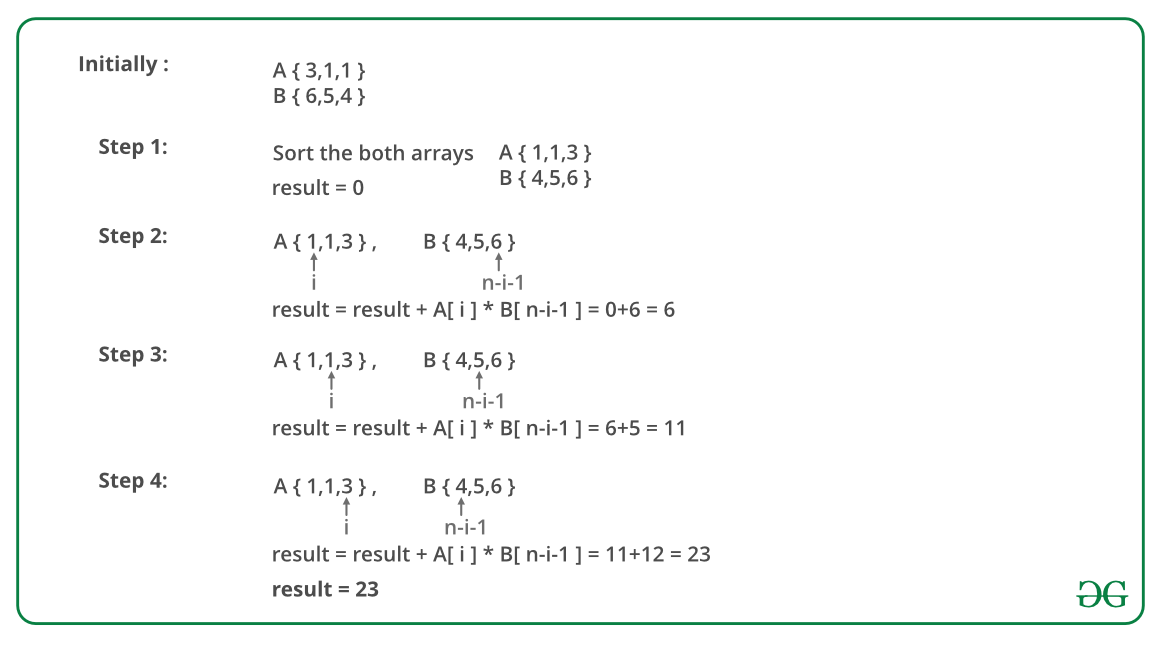Related Articles

# Minimize the sum of product of two arrays with permutations allowed

• Difficulty Level : Easy
• Last Updated : 25 Mar, 2021

Given two arrays, A and B, of equal size n, the task is to find the minimum value of A * B + A * B +…+ A[n-1] * B[n-1]. Shuffling of elements of arrays A and B is allowed.

Examples :

Attention reader! Don’t stop learning now. Get hold of all the important DSA concepts with the DSA Self Paced Course at a student-friendly price and become industry ready.  To complete your preparation from learning a language to DS Algo and many more,  please refer Complete Interview Preparation Course.

In case you wish to attend live classes with experts, please refer DSA Live Classes for Working Professionals and Competitive Programming Live for Students.

```Input : A[] = {3, 1, 1} and B[] = {6, 5, 4}.
Output : 23
Minimum value of S = 1*6 + 1*5 + 3*4 = 23.

Input : A[] = { 6, 1, 9, 5, 4 } and B[] = { 3, 4, 8, 2, 4 }
Output : 80.
Minimum value of S = 1*8 + 4*4 + 5*4 + 6*3 + 9*2 = 80.```

The idea is to multiply minimum element of one array to maximum element of another array. Algorithm to solve this problem:

1. Sort both the arrays A and B.
2. Traverse the array and for each element, multiply A[i] and B[n – i – 1] and add to the total.

Note: We are adding multiplication of elements which can lead to overflow conditions.

Below image is an illustration of the above approach:Below is the implementation of the above approach:

## C++

 `// C++ program to calculate minimum sum of product``// of two arrays.``#include ``using` `namespace` `std;``  ` `// Returns minimum sum of product of two arrays``// with permutations allowed``long` `long` `int` `minValue(``int` `A[], ``int` `B[], ``int` `n)``{``    ``// Sort A and B so that minimum and maximum``    ``// value can easily be fetched.``    ``sort(A, A + n);``    ``sort(B, B + n);``  ` `    ``// Multiplying minimum value of A and maximum``    ``// value of B``    ``long` `long` `int` `result = 0;``    ``for` `(``int` `i = 0; i < n; i++)``        ``result += (A[i] * B[n - i - 1]);``  ` `    ``return` `result;``}``  ` `// Driven Code``int` `main()``{``    ``int` `A[] = { 3, 1, 1 };``    ``int` `B[] = { 6, 5, 4 };``    ``int` `n = ``sizeof``(A) / ``sizeof``(A);``    ``cout << minValue(A, B, n) << endl;``    ``return` `0;``}`

## Java

 `// Java program to calculate minimum``// sum of product of two arrays.``import` `java.io.*;``import` `java.util.*;` `class` `GFG {` `    ``// Returns minimum sum of product of two arrays``    ``// with permutations allowed``    ``static` `long` `minValue(``int` `A[], ``int` `B[], ``int` `n)``    ``{``        ``// Sort A and B so that minimum and maximum``        ``// value can easily be fetched.``        ``Arrays.sort(A);``        ``Arrays.sort(B);` `        ``// Multiplying minimum value of A``        ``// and maximum value of B``        ``long` `result = ``0``;``        ``for` `(``int` `i = ``0``; i < n; i++)``            ``result += (A[i] * B[n - i - ``1``]);` `        ``return` `result;``    ``}` `    ``// Driven Code``    ``public` `static` `void` `main(String[] args)``    ``{``        ``int` `A[] = { ``3``, ``1``, ``1` `};``        ``int` `B[] = { ``6``, ``5``, ``4` `};``        ``int` `n = A.length;``        ``;``        ``System.out.println(minValue(A, B, n));``    ``}``}` `// This code is contributed by vt_m`

## Python

 `# Python program to calculate minimum sum of product``# of two arrays.` `# Returns minimum sum of product of two arrays``# with permutations allowed`  `def` `minValue(A, B, n):` `    ``# Sort A and B so that minimum and maximum``    ``# value can easily be fetched.``    ``A.sort()``    ``B.sort()` `    ``# Multiplying minimum value of A and maximum``    ``# value of B``    ``result ``=` `0``    ``for` `i ``in` `range``(n):``        ``result ``+``=` `(A[i] ``*` `B[n ``-` `i ``-` `1``])` `    ``return` `result`  `# Driven Program``A ``=` `[``3``, ``1``, ``1``]``B ``=` `[``6``, ``5``, ``4``]``n ``=` `len``(A)``print` `minValue(A, B, n)` `# Contributed by: Afzal Ansari`

## C#

 `// C# program to calculate minimum``// sum of product of two arrays.``using` `System;` `class` `GFG {` `    ``// Returns minimum sum of product``    ``// of two arrays with permutations``    ``// allowed``    ``static` `long` `minValue(``int``[] a, ``int``[] b,``                                   ``int` `n)``    ``{``        ` `        ``// Sort A and B so that minimum``        ``// and maximum value can easily``        ``// be fetched.``        ``Array.Sort(a);``        ``Array.Sort(b);` `        ``// Multiplying minimum value of``        ``// A and maximum value of B``        ``long` `result = 0;``        ` `        ``for` `(``int` `i = 0; i < n; i++)``            ``result += (a[i] * b[n - i - 1]);` `        ``return` `result;``    ``}` `    ``// Driven Code``    ``public` `static` `void` `Main()``    ``{``        ` `        ``int``[] a = { 3, 1, 1 };``        ``int``[] b = { 6, 5, 4 };``        ``int` `n = a.Length;``        ` `        ``Console.Write(minValue(a, b, n));``    ``}``}` `// This code is contributed by nitin mittal.`

## PHP

 ``

## Javascript

 ``

Output :

`23`

Time Complexity : O(n log n).

This article is contributed by Anuj Chauhan(anuj0503). If you like GeeksforGeeks and would like to contribute, you can also write an article using contribute.geeksforgeeks.org or mail your article to contribute@geeksforgeeks.org. See your article appearing on the GeeksforGeeks main page and help other Geeks.